# Does Maxwell Tensor include Lorentz Forces ?

## Electromagnetic problem

An analytical case is presented in order to show that the airgap Maxwell Tensor method is taking into account the Lorentz forces (sometimes called Laplace forces).Let us have a straight electrical wire surrounded by the void and traversed by a current. The wire is oriented toward z-axis, as illustrated in the previous figure.The magnetic flux generated by the wire can be expressed in the polar referential as:This wire is immersed in an external homogeneous electromagnetic fieldsuch that the total electromagnetic field is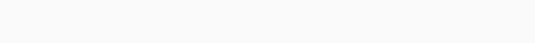which can be written as: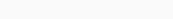## Lorentz force

According to the Lorentz force acting on a section L of the wire is given by: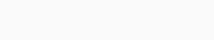## Maxwell Tensor

According to the Maxwell Tensor method, the total magnetic force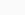can be computed by integrating the Maxwell stress Tensor on a closed cylinder of height L and radius R: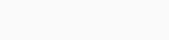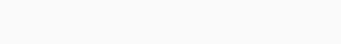such that the total force in the x-direction is: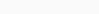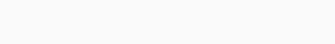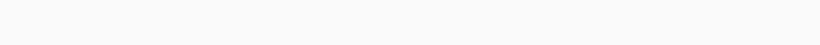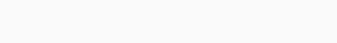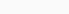Following the same method in the y-direction leads to: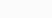## Conclusion

Then the Lorentz’s forces and the Maxwell forces are equal in this case: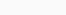It confirms that the integration of Maxwell stress tensor on a closed surface is more general than the Lorentz force method.